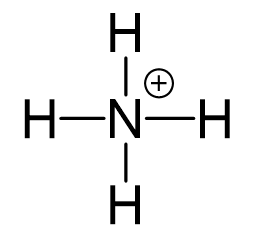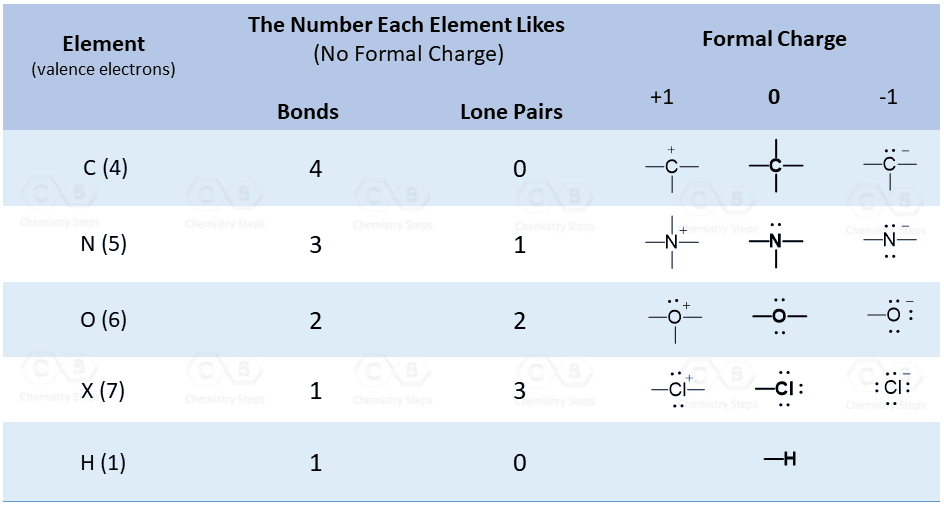## Examples

Nitrogen is the central atom, so we can draw a preliminary skeletal structure:There is a positive charge, so to determine the number of electrons, we subtract 1 from the total number of valence electrons:

5 + 4 – 1 = 8 electrons

All the electrons are used to make the 4 covalent bonds, and the positive charge is on the nitrogen:Remember, the formula for calculating the formal charge:

FC= V – (N + B)

Where:

V – number of valence electrons
N – number of nonbonding electrons
B – number of bonds

So, for the nitrogen it would be: 5 – (0 + 4) = +1

Try memorizing the bonding and formal charge patterns to make this process easier:For the geometry, the nitrogen has 4 atoms and no lone pairs, therefore, both geometries are tetrahedral.Steric number 4 corresponds to sp3-hybridization where the idealized bond angles are 109.5o